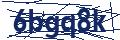Call / Whatsapp# Do you have the same implied volatility for the two options on the same underlying? If not (in which case it is referred to as volatility smile), what might be able to explain the differences? (Hint: refer to the chapter on volatility smile).

Answer the following questions given the following call
option prices on Google (GOOG) and on Apple (APPL). Note that these are actual
option prices on 2/21/13 and these contracts have 60 days till expiration. The
2-month T-bill rate is about 4.75%. Show all work.

 OPTION STRIKE EXP VOL LAST GOOG 800 APR 378 28.20 S=795.53 690 APR 53 101.57 APPL 450 APR 530 18.55 S=446.06 480 APR 856 7.81

Part One

1. Estimate the theoretical option values for the call on
GOOG with K =800 and for the call on APPL with K = 450 using the
Black-Scholes-Merton and Binomial Models program (available under doc sharing).
You can also use the following website to calculate the option prices and
implied volatility

www.option-price.com

2. When estimating
the option values assume various standard deviations of returns of 10%, 15%,
20%, … up to 100% or until you find the theoretical option value is close to
the actual one.

3. Draw a graph showing the relationship between standard
deviations and option values.

4. Based on the
graph, what does the actual option value imply about the expected future
standard deviation (volatility)? Which option has higher implied volatility and
is it surprising?

Part Two

1. Estimate the historical standard deviation of GOOG*.

2. Compare the implied standard deviation with the
historical standard deviation.

3. What can you infer from the difference, if any, between
the two numbers?

Part three

1. Compute the implied volatility for all options.

2. Do you have the same implied volatility for the two
options on the same underlying? If not (in which case it is referred to as
volatility smile), what might be able to explain the differences? (Hint: refer
to the chapter on volatility smile).

_____________________________________________________________________________________

*: How to estimate the historical standard deviation:

1. You need to obtain historical stock prices. I recommend
using Yahoo Finance .yahoo.com/”>/(enter symbol)–chart-http://finance.yahoo.com (daily or weekly) historical
quotes

2. Calculate stock return

Return on day T+1
= (stock price on day T+1 – stock price on day T)/ stock price on day T

Rt+1= (St+1– St)
/ St

·
For simplicity we can ignore dividends, since
GOOG pays no dividend.

·
A minimum of 30 returns is required—I am not
requiring an exact time period or the length of time period, so that your
answers will be slightly different and answers identical to those of other
students might receive point deductions.

3. The standard deviation (SD) of Stock Returns can then be
estimated using EXCEL function STDEV.S(range of return data). Further, the SD
needs to be annual standard deviation. If you use daily returns, the SD you
obtain is a daily one; you need to transform it to Annual SD = Daily SD * (365)0.5. For weekly data, Annual SD = Weekly SD * (52)0.

## Price: £ 45

100% Plagiarism Free & Custom Written, Tailored to your instructions